Next: Summary of results Up: Lunar motion Previous: Evection in latitude

# Reduction to ecliptic

Finally, let us calculate the remaining terms in the solution of the lunar equations of motion.

According to Equation (11.153),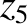(11.321)

It follows from Equations (11.209), (11.211), (11.290), and (11.315) that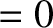(11.322)

According to Equation (11.154),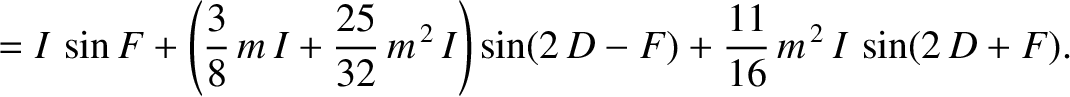(11.323)

It follows from Equations (11.209), (11.212), (11.290), and (11.315) that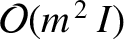(11.324)

According to Equation (11.127),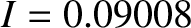(11.325)

where use has been made of Equation (11.315). Equation (11.189) yields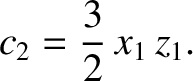(11.326)

Finally, according to Equations (11.130) and (11.142),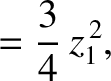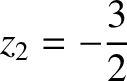(11.327) and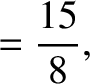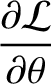(11.328)

where use has been made of Equation (11.315). Hence, Equations (11.192), (11.193), and (11.196) yield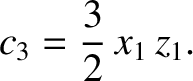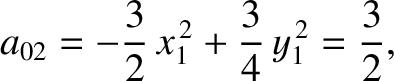(11.329) and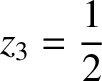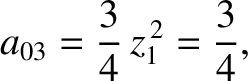(11.330)

It follows from Equations (11.122)-(11.124), (11.159), (11.162), (11.174), (11.185), and (11.186), as well as the previous expressions for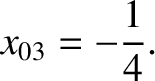,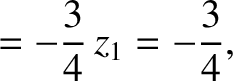,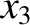,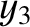, and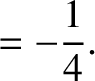, that the net perturbation to the lunar orbit due to the remaining terms in the solution of the lunar equations of motion is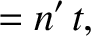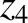(11.331)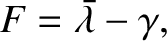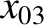(11.332) and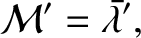(11.333)

These expressions are accurate toand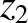.

All of the terms on the right-hand sides of Equations (11.332) and (11.333) are Keplerian in origin (i.e., they are independent of the perturbing influence of the Sun). The term on the right-hand side of Equation (11.332) is due to the slight inclination of the lunar orbit to the ecliptic plane, and is known as the reduction to the ecliptic.Next: Summary of results Up: Lunar motion Previous: Evection in latitude
Richard Fitzpatrick 2016-03-31